TitleBeginning Algebra
Answer/Discussion to Practice Problems
Tutorial 31: Practice Test on Tutorials 26 - 30

 Problem 1a: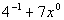Answer: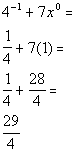Problem 1b: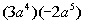Answer: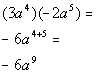Problem 1c: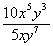Answer: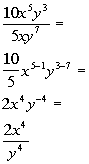Problem 1d: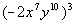Answer: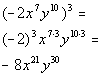Problem 1e: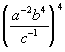Answer: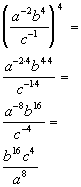Problem 1f: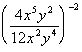Answer: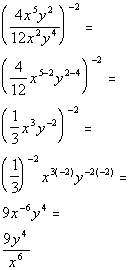Problem 2a:

 73,200,000Answer: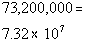Problem 3a: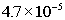Answer: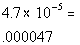Problem 4a: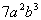Answer: Since the degree is the sum of the variable exponents and it looks like we have a 2 and a 3 as our exponents, the degree would have to be 2 + 3 = 5.

 Problem 4b:

 -8Answer: Since this is a constant term, it's degree is 0.

 Problem 5a: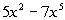Answer: Since the degree of the polynomial is the highest degree of all the terms, it looks like the degree is 5. Since there are two terms, this is a binomial.

 Problem 5b: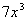Answer: Since the degree of the polynomial is the highest degree of all the terms, it looks like the degree is 3. Since there is one term, this is a monomial.

 Problem 5c: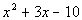Answer: Since the degree of the polynomial is the highest degree of all the terms, it looks like the degree is 2. Since there are two terms, this is a trinomial.

 Problem 6a: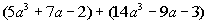Answer: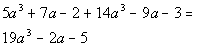Problem 6b: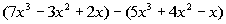Answer: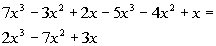Problem 7a: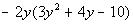Answer: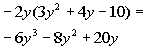Problem 7b: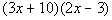Answer: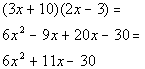Problem 7c: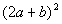Answer: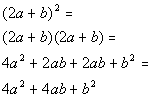Problem 7d: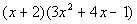Answer: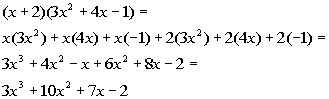Problem 8a: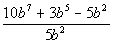Answer: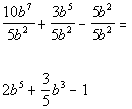Problem 8b: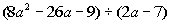Answer: Step 1: Set up the long division  AND Step 2: Divide 1st term of divisor by first term of dividend to get first term of the quotient AND Step 3:  Take the term found in step 1 and multiply it times the divisor AND Step 4:  Subtract this from the line above AND Step 5:  Repeat until done AND Step 6: Write out the answer.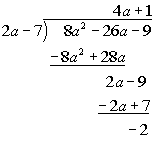Final answer: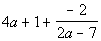Last revised on August 2, 2011 by Kim Seward.
All contents copyright (C) 2001 - 2011, WTAMU and Kim Seward. All rights reserved.